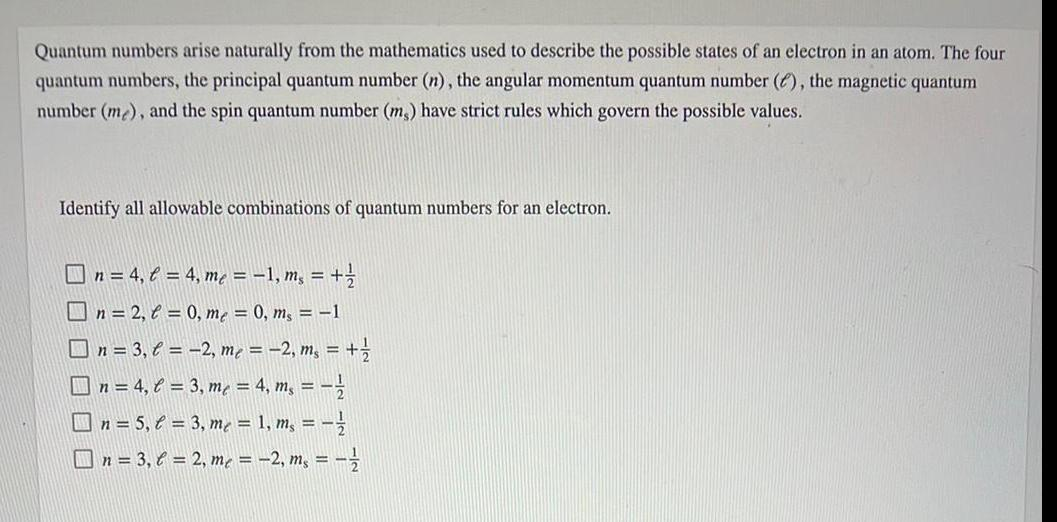Question:

# Quantum numbers arise naturally from the mathematics used to

Last updated: 8/7/2022Quantum numbers arise naturally from the mathematics used to describe the possible states of an electron in an atom. The four quantum numbers, the principal quantum number (n), the angular momentum quantum number (), the magnetic quantum number (me), and the spin quantum number (ms) have strict rules which govern the possible values. Identify all allowable combinations of quantum numbers for an electron. n = 4₁ l = 4, m₁ = − 1, m, = + 1/ n=2, = 0, me = 0, ms = 1 n = 3, l = -2, me = -2, ms = + 1/2 n = 4, C = 3, m² = 4, m₁ = = ²/ n = 5₁ € = 3, me = 1, ms == // n = 3,6 = 2, me = -2, ms = 1/2